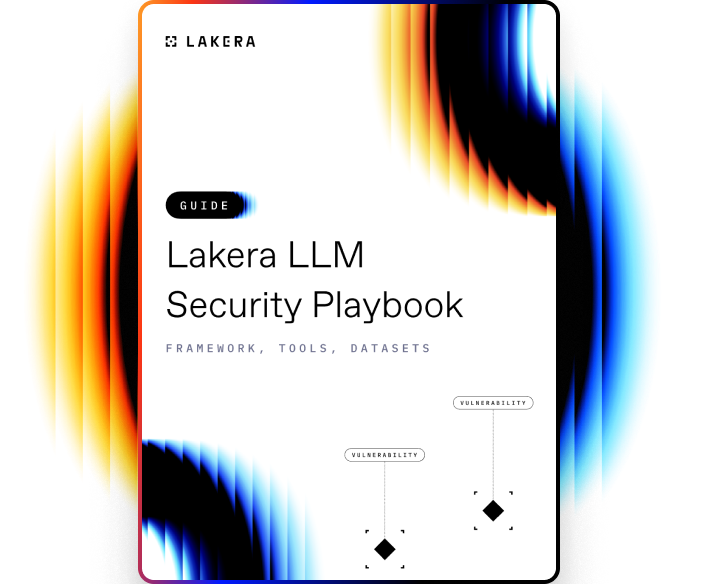VOCABULARY

# K-means

K-means is a popular unsupervised machine learning algorithm primarily used for data clustering. It segregates data into k different clusters based on the attributes and characteristics of the data points. The goal of the K-means algorithm is to minimize the distance between the data points and their corresponding cluster centroid, where each cluster's centroid is nothing but the mean of the data points in the cluster.

## How K-means works

The K-means algorithm works in an iterative manner. Here are the basic steps:

1. Initialization: Start by choosing 'k' random points as the initial centroids.
2. Assignment: Assign each data point to the nearest centroid. The measure of distance can be Euclidean, Manhattan, Cosine, etc. The data points closest to a centroid will form a cluster.
3. Update: Once all data points have been assigned to clusters, compute the new centroid of each cluster. The new centroid is the mean of all points in the cluster.
4. Iteration: Repeat the assignment and update steps until the centroid positions do not change or the change is below a threshold limit, or until a maximum number of iterations is reached.

The result is 'k' clusters with minimized within-cluster variance. However, it is crucial to note that the K-means algorithm may converge to a local minimum, which means the outcome can differ based on the initial selection of centroids. A common solution to this problem is running K-means multiple times with different initial values and choosing the result with the lowest variance.Learn how to protect against the most common LLM vulnerabilities

Download this guide to delve into the most common LLM security risks and ways to mitigate them.

Related termsActivate
untouchable mode.